# Parallel Force and Heat Balance

Let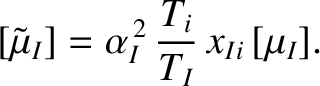(A.69)

The requirement of equilibrium force and heat balance parallel to the magnetic field (see Sections 2.18 and 2.19) leads us to define four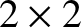dimensionless ion matrices,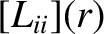,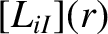,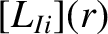, and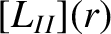, where [7,9]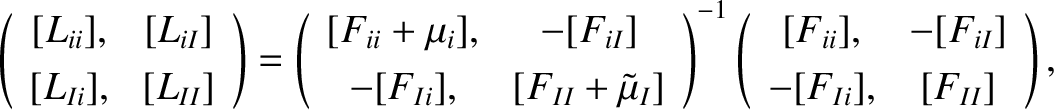(A.70)

and thedimensionless electron matrices,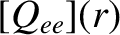,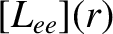,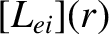, and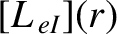, where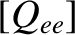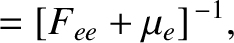(A.71)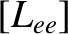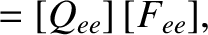(A.72)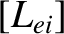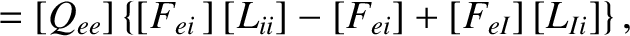(A.73)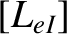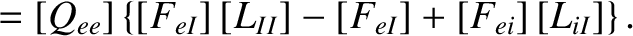(A.74)